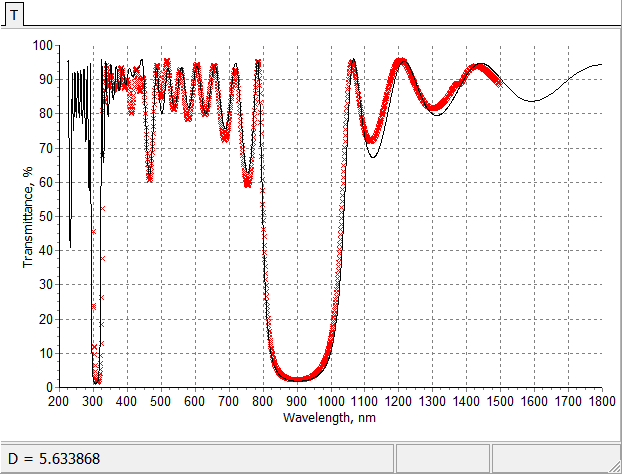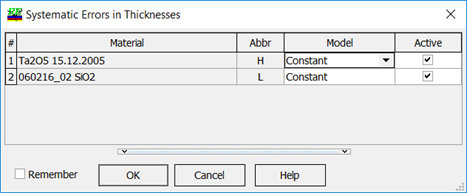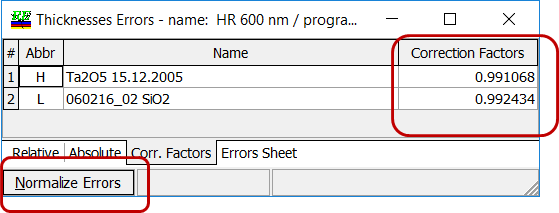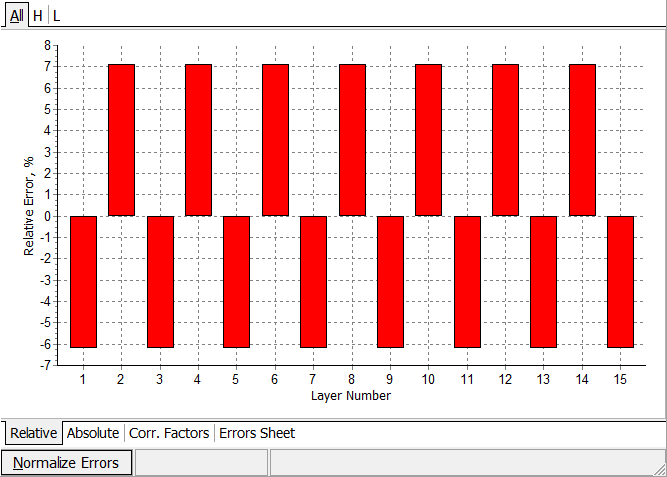### Systematic Errors

 Systematic errors in measurement data may be caused by various factors, for example:  signal drifts in the registration circuits of spectrophotometric devices; inaccurate calibration of monitoring devices;  vacuum shift; inaccuracy of tooling factors for witness glass. These factors may result in the shift of measured transmittance/reflectance data as a whole or in the scaling them by a factor that differs from 1.  From a mathematical point of view, in the course of Systematic errors procedure only two unknown parameters are searched for. Optical coating model in this case can be described by vector $$X$$: $X=d_1+\Delta_H, d_2+\Delta_L,...,d_m+\Delta_H(L)$Fitting before and after application of systematic errors model (put the mouse on and out of picture)Discrepancy function is optimized with respect to systematic shifts $$\Delta_H, \Delta_L$$: $DF^2(\Delta_H, \Delta_L)\rightarrow \min$ OptiRE allows you to represent any errors as a sum of systematic errors and pure random errors with average value with respect to any layer material equal to zero (Normalize Errors); Correction factors present systematic components of the ratios of actual thicknesses to theoretical ones (useful for adjusting the deposition process)The results of the procedure are presented as Relative, Absolute errors or correction factors for layers of active materials. Systematic errors procedure can be easily run from an external software with the help of COM Automation tool.